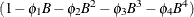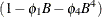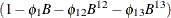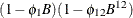# The ARIMA Procedure

### Subset, Seasonal, and Factored ARMA Models

Subsections:

The simplest way to specify an ARMA model is to give the order of the AR and MA parts with the P= and Q= options. When you do this, the model has parameters for the AR and MA parts for all lags through the order specified. However, you can control the form of the ARIMA model exactly as shown in the following section.

#### Subset Models

You can control which lags have parameters by specifying the P= or Q= option as a list of lags in parentheses. A model that includes parameters for only some lags is sometimes called a subset or additive model. For example, consider the following two ESTIMATE statements:

      identify var=sales;
estimate p=4;
estimate p=(1 4);


Both specify AR(4) models, but the first has parameters for lags 1, 2, 3, and 4, while the second has parameters for lags 1 and 4, with the coefficients for lags 2 and 3 constrained to 0. The mathematical form of the autoregressive models produced by these two specifications is shown in Table 7.1.

Table 7.1: Saturated versus Subset Models

Option

Autoregressive Operator

P=4P=(1 4)#### Seasonal Models

One particularly useful kind of subset model is a seasonal model. When the response series has a seasonal pattern, the values of the series at the same time of year in previous years can be important for modeling the series. For example, if the series SALES is observed monthly, the statements

      identify var=sales;
estimate p=(12);


model SALES as an average value plus some fraction of its deviation from this average value a year ago, plus a random error. Although this is an AR(12) model, it has only one autoregressive parameter.

#### Factored Models

A factored model (also referred to as a multiplicative model) represents the ARIMA model as a product of simpler ARIMA models. For example, you might model SALES as a combination of an AR(1) process that reflects short term dependencies and an AR(12) model that reflects the seasonal pattern.

It might seem that the way to do this is with the option P=(1 12), but the AR(1) process also operates in past years; you really need autoregressive parameters at lags 1, 12, and 13. You can specify a subset model with separate parameters at these lags, or you can specify a factored model that represents the model as the product of an AR(1) model and an AR(12) model. Consider the following two ESTIMATE statements:

      identify var=sales;
estimate p=(1 12 13);
estimate p=(1)(12);


The mathematical form of the autoregressive models produced by these two specifications are shown in Table 7.2.

Table 7.2: Subset versus Factored Models

Option

Autoregressive Operator

P=(1 12 13)P=(1)(12)Both models fit by these two ESTIMATE statements predict SALES from its values 1, 12, and 13 periods ago, but they use different parameterizations. The first model has three parameters, whose meanings may be hard to interpret.

The factored specification P=(1)(12) represents the model as the product of two different AR models. It has only two parameters: one that corresponds to recent effects and one that represents seasonal effects. Thus the factored model is more parsimonious, and its parameter estimates are more clearly interpretable.